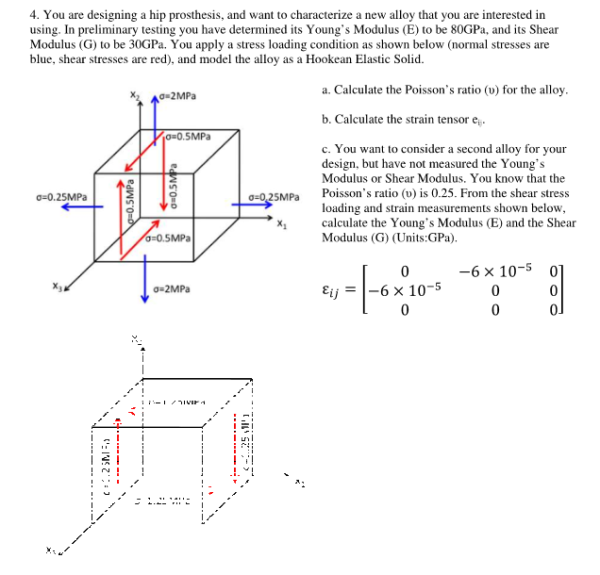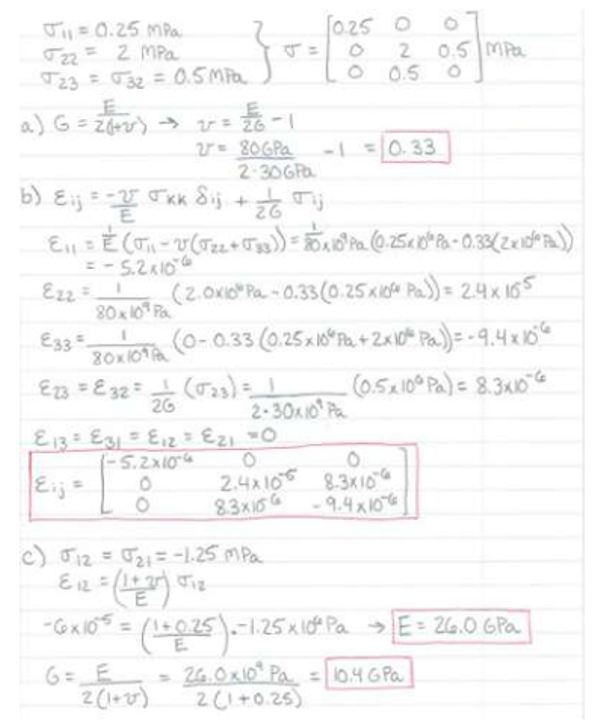You are designing a hip prosthesis, and want to characterize a new alloy that you are interested in using. In preliminary testing you have determined its Young's Modulus E to be 80 GPa, and its Shear Modulus G to be 30 GPa. you apply a stress loading condition as shown below, and model the alloy as a Hookean Elastic Solid. a) Calculate the Poisson's ratio for the alloy. b) Calculate teh strain tensor. c) You want to consider a second alloy for your design, but have not measured the Young's Modulus or Shear Modulus. You know that the Poisson's ratio is 0.25. From the shear stress loading and strain measurements shown below, calculate the Young's Modulus E and the Shear Modulus G.You are designing a hip prosthesis, and want to characterize a new alloy that you are interested in using. In preliminary testing you have determined its Young's Modulus E to be 80 GPa, and its Shear Modulus G to be 30 GPa. you apply a stress loading condition as shown below, and model the alloy as a Hookean Elastic Solid. a) Calculate the Poisson's ratio for the alloy. b) Calculate teh strain tensor. c) You want to consider a second alloy for your design, but have not measured the Young's Modulus or Shear Modulus. You know that the Poisson's ratio is 0.25. From the shear stress loading and strain measurements shown below, calculate the Young's Modulus E and the Shear Modulus G.

biomechanics page 1 biomechanics biomechanics biomechanics biomechanics biomechanics biomechanics biomechanics biomechanics page 2